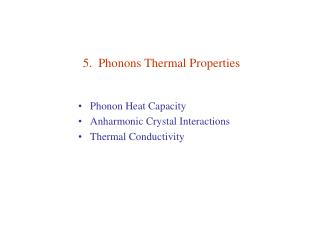DownloadDownload Presentation5. Phonons Thermal Properties

# 5. Phonons Thermal Properties

Download Presentation## 5. Phonons Thermal Properties

- - - - - - - - - - - - - - - - - - - - - - - - - - - E N D - - - - - - - - - - - - - - - - - - - - - - - - - - -
##### Presentation Transcript

1. 5. Phonons Thermal Properties • Phonon Heat Capacity • Anharmonic Crystal Interactions • Thermal Conductivity

2. Phonon Heat Capacity • Planck Distribution • Normal Mode Enumeration • Density of States in One Dimension • Density of States in Three Dimensions • Debye Model for Density of States • Debye T3 Law • Einstein Model of the Density of States • General Result for D(ω)

3. where = Thermal expansivity = isothermal compressibility = 1/ B B = Bulk modulus α = linear (1-D) thermal expansivity

4. Lattice heat capacity: p = polarization Planck distribution:

5. Planck Distribution System at constant T Canonical ensemble : Boltzmann factor For a set of identical harmonic oscillators Nn = number of oscillators in the nth excited state when system is in thermal equilibrium Probability of an oscillator in the nth excited state: Occupation number:

6. Normal Mode Enumeration

7. Density of States in One Dimension Fixed boundary problem of N+1 particles. N = 10 → with Number of allowed K for non-stationary solutions is N–1 = Number of mobile atoms Polarization p : 1 long, 2 trans

8. Periodic boundary problem of N particles N = 8 → with → Number of allowed K for non-stationary solutions is N = Number of mobile atoms

9. fixed B.C. → Periodic B.C. →

10. Density of States in Three Dimensions Periodic B.C. ; N 3 cells in cube of side L → density of states in K-space is Number of modes per polarization lying between ωand ω + d ωis → density of states in ω-space is For isotropic materials, →

11. Debye Model for Density of States Debye model: v velocity of sound (for a given type of polarization) For a crystal of N primitive cells: →  Debye frequency

12. Thermal (vibrational) energy  Debye integrals: See Ex on Zeta functions, Arfken where θ Debye temperature for each acoustic branch

13. Debye model

14. Debye T3 Law For low T, xD →  : → for each acoustic branch To account for all 3 acoustic branches, we set and so that Good for T < θ /50

15. Solid Ar, θ = 92K

16. c.f. Table for Bulk Moduli, Chap 3, p.52

17. Qualitative Explanation of the T3 Law Of the 3N modes, only a fraction (KT /KD ) 3 = ( T / θ)3 is excited. →

18. Einstein Model of the Density of States N oscillators of freq ω0. Diamond Classical statistical mechanics: Dulong-Petit valueCV = 3NkB

19. General Result for D(ω) Si Debye solid vg ~ 0 Van Hove Singularities

20. Anharmonic Crystal Interactions • Harmonic (Linear) Waves: • Normal modes do not decay. • Normal modes do not interact. • No thermal expansion. • Adiabatic & isothermal elastic constants are equal. • Elastic constants are independent of P and T. • C → constant for T > θ . Deviation from harmonic behavior → Anharmonic effects

21. Thermal Expansion 1-D anharmonic potential: c = 1 g = .2 f = .05 Boltzmann distribution High T: → Thermal Expansion

22. Lattice constant of solid argon

23. Thermal Conductivity For phonons, JQ = JU . Heat current density: κ = Thermal conductivity coefficient • Key features of kinetic theory (see L.E.Reichl, “A Modern Course of Statistical Mechanics”, §13.4 ): • Quantities not conserved in particle collisions are quickly thermalized to (global) equilibrium values. (e.g., velocity directions & magnitudes ) • Conserved quantities can remain out of global equilibrium (e.g., stay in local equilibrium. They get transported spatially in the presence of a “gradient”. • MFP is determined by collisions that do not conserve the total momenta of particles. Net amount of A(z) transported across the x-y plane at z0in the +z direction per unit area per unit time: Δz = distance above/below plane at which particle suffered last collision. n = particle density, l = mean free path, a = some constant. bA = 1/3 is determined from self diffusion

24. For heat conduction, we set where c = heat capacity per particle = sound velocity C = nc = heat capacity

25. Thermal Resistivity of Phonon Gas Harmonic phonons: mfp l determined by collisions with boundaries & imperfections. Anharmonic phonons: only U-processes contribute.

26. Gas: Elastic collisions. No T required. κ =  Gas: No net mass flow. Inelastic collisions with walls sets up T & n gradients. Finite κ. Crystal: N-processes only. κ = . Crystal: U-processes. Finiteκ.

27. Umklapp Processes 2-D square lattice Normal processes: Umklapp processes: Energy is conserved: Condition: T > θ: all modes excited → no distinction between N- & U- processes → l 1/T. T < θ: probability of U- processes & hence l1 exp(–θ /2T).

28. Imperfections Low T → umklapp processes negligible. Geometric effect dominates. Size effect: l > D = smallest dimension of specimen. Dielectric crystals can have thermal conductivities comparable to those of metals. Sapphire (Al2O3): κ ~ 200W cm–1 K–1 at 30K. Cu: max κ ~ 100W cm–1 K–1. Metalic Ga: κ ~ 845W cm–1 K–1 at 1.8K. ( Electronic contributions dominate in metals. ) Highly purified c-NaF

29. Isotope effect on Ge. Enriched: 96% Ge74. Normal: 20% Ge70 , 8% Ge73 , 37% Ge74 , 8% Ge76.